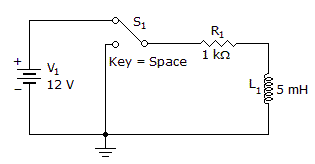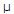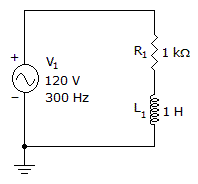# Electronics - Inductors

### Exercise :: Inductors - General Questions

36.

How long until the current is at a maximum?A. 0s B. 5s C. 15s D. 25s

Answer: Option B

Explanation:

No answer description available for this question. Let us discuss.

37.

Find VR and VL.A. VR = 41.6 V, VL = 78.4 V B. VR = 48 V, VL = 110 V C. VR = 56 V, VL = 106 V D. VR = 60 V, VL = 60 V

Answer: Option C

Explanation:

No answer description available for this question. Let us discuss.

38.

If 360 Vac is the output of a 300-turn secondary winding, what is the input voltage when the primary winding has 150 turns?

 A. 2.4 Vac B. 150 Vac C. 180 Vac D. 720 Vac

Answer: Option C

Explanation:

No answer description available for this question. Let us discuss.

39.

When a magnet enters a coil and a voltage is induced, the process is known as:

 A. an alternation B. self-inductance C. counter emf D. flux linkage

Answer: Option D

Explanation:

No answer description available for this question. Let us discuss.

40.

What term is used to describe the action of magnetic flux from one circuit that cuts across the conductors of another circuit?

 A. self-inductance B. rectification C. mutual inductance D. magnetism

Answer: Option C

Explanation:

No answer description available for this question. Let us discuss.

41.

Reactance in an inductive circuit will:

 A. increase with frequency B. decrease with frequency C. be independent from frequency D. depend on the value of XC

Answer: Option A

Explanation:

No answer description available for this question. Let us discuss.

42.

A turns ratio of 5 for a transformer implies:

 A. more primary turns than secondary turns B. more secondary current than primary current C. larger source impedance than load impedance D. more secondary turns than primary turns

Answer: Option D

Explanation:

No answer description available for this question. Let us discuss.

#### Current Affairs 2021

Interview Questions and Answers